## UGC NET CS 2015 June Paper-3

 Question 1
For the 8 - bit word 00111001, the check bits stored with it would be 0111. Suppose when the wo​rd is read from memory, the check bits are calculated to be 1101. What is the data word that was read from memory?
 A 10011001 B 00011001 C 00111000 D 11000110
Digital-Logic-Design       Number-Systems
Question 1 Explanation:
Here, the check bits stored along with data are 0111 and the check bits calculated at receiver side are 1101. Both check bits are not same is the indication of error bit in data whose position can be calculated by performing the EX-OR operation.So data bits are:
00011001
 Question 2
Consider a 32 - bit microprocessor, with a 16 - bit external data bus, driven by an 8 MHz input clock. Assume that this microprocessor has a bus cycle whose minimum duration equals four input clock cycles. What is the maximum data transfer rate for this microprocessor?
 A 8 × 10​ 6​ bytes/sec B 4 × 10​ 6​ bytes/sec C 16 × 10​ 6​ bytes/sec D 4 × 10​ 9​ bytes/sec
Computer-Organization       Microprocessor
Question 2 Explanation:Question 3
The RST 7 instruction in 8085 microprocessor is equivalent to:
 A CALL 0010 H B CALL 0034 H C CALL 0038 H D CALL 003C H
Computer-Organization       Microprocessor
Question 3 Explanation: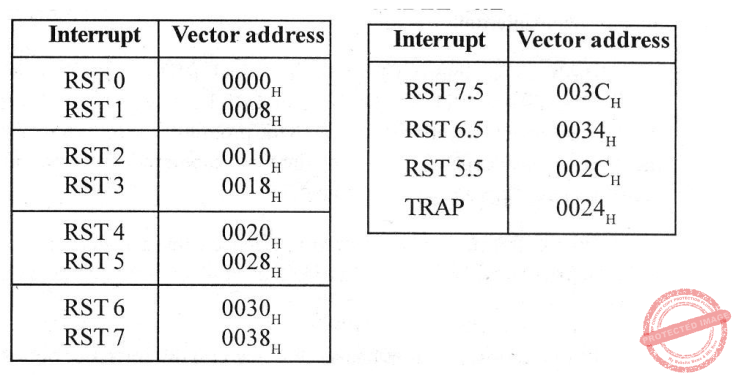Question 4
The equivalent hexadecimal notation for octal number 2550276 is:
Digital-Logic-Design       Number-Systems
Question 4 Explanation: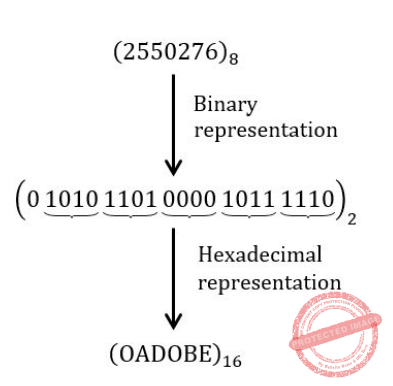Question 5
The CPU of a system having 1 MIPS execution rate needs 4 machine cycles on an average for executing an instruction. The fifty percent of the cycles use memory bus. A memory read/ write employs one machine cycle. For execution of the programs, the system utilizes 90 percent of the CPU time. For block data transfer, an IO device is attached to the system while CPU executes the background programs continuously. What is the maximum IO data transfer rate if programmed IO data transfer technique is used?
 A 500 Kbytes/sec B 2.2 Mbytes/sec C 125 Kbytes/sec D 250 Kbytes/sec
Computer-Organization       Microprogrammed-Control-Unit
Question 5 Explanation:
Given, CPU speed 10 6 instruction/sec
1 CPU instruction = 4 machine cycles
CPU utilization = 90%
Programmed IO: each byte transfer requires 4 cycles (instructions), in status, check status branch, R/W data in memory maximum data transfer rate,Question 6
The number of flip-flops required to design a modulo - 272 counter is:
 A 8 B 9 C 27 D 11
Digital-Logic-Design       Sequential-Circuits
Question 6 Explanation:
If we have “n” flip-flops then total number of combinations possible is 2​ n​ .
So total number of states possible is 2​ n​ i.e. MOD 2​ n​ .
Here 2​ n​ = 272
So n = log​ 2​ 272
n = 9
 Question 7
Let E​ 1​ and E​ 2​ be two entities in E-R diagram with simple single valued attributes. R1 and R​ 2​ are two relationships between E​ 1​ and E​ 2​ where R​ 1​ is one - many and R​ 2​ is many - many. R​ 1​ and R​ 2​ do not have any attributes of their own. How many minimum number of tables are required to represent this situation in the Relational Model?
 A 4 B 3 C 2 D 1
Database-Management-System       ER-Model
Question 7 Explanation:Question 8
The STUDENT information in a university stored in the relation STUDENT (Name, SEX, Marks, DEPT_Name) Consider the following SQL Query SELECT DEPT_Name from STUDENT where SEX = 'M' group by DEPT_Name having avg (Marks)>SELECT avg (Marks) from STUDENT. It Returns the Name of the Department for which:
 A The Average marks of Male students is more than the average marks of students in the same Department B The average marks of male students is more than the average marks of students in the University C The average marks of male students is more than the average marks of male students in the University D The average marks of students is more than the average marks of male students in the University
Database-Management-System       SQL
Question 8 Explanation:
The query is co-related( same table is used in outer and inner query) so compare each entry of Student table 1 with each entry of Student table 2.⇒ Consider this table for outer query i.e., LHS query of “>” symbol and here find avg. marks of a department because of group by constraint
i.e., avg. marks for CSE = 87.5
avg. marks for IT = 90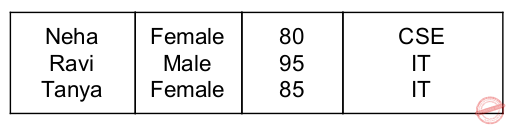⇒ Consider this table for inner query i.e., RHS query of “>” symbol and here find avg. of total marks
i.e., avg. marks = 88.7
⇓ O/P of given query is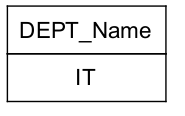Question 9
Select the 'False' statement from the following statements about Normal Forms:
 A Lossless preserving decomposition into 3NF is always possible B Lossless preserving decomposition into BCNF is always possible C Any Relation with two attributes is in BCNF D BCNF is stronger than 3NF
Database-Management-System       Normalization
Question 9 Explanation:
Option(A) is true because 3NF preserves lossless decomposition and functional dependency.
Option(B) is false because BCNF ensures lossless decomposition but do not preserves functional dependency.
Option(C) is true because Any Relation with two attributes is in BCNF
Option(D) is true because BCNF ensure less number of duplicate values in a relation than 3NF.
 Question 10
The Relation Vendor Order (V_no, V_ord_no, V_name, Qty_sup, unit_price) is in 2NF because:
 A Non_key attribute V_name is dependent on V_no which is part of composite key B Non_key attribute V_name is dependent on Qty_sup C Key attribute Qty_sup is dependent on primary_key unit price D Key attribute V_ord_no is dependent on primary_key unit price E None of the above
Database-Management-System       Normalization
Question 10 Explanation:
A relation is in 2NF if there is no partial dependency in a relation. A relation will be in 2NF if the LHS of a functional dependency is either a primary key or a non- key attribute or if all the attributes of a relation are prime attributes.
Option(A) is not correct because Non_key attribute V_name is dependent on V_no which is part of composite key i.e there is a partial dependency exists because of V_no.
Option(B) is also not correct because there are chances of having a partial dependency because Qty_sup could be a prime attribute.
Option(C) and Option(D) are correct because in both the options Key attributes depend upon primary key.
 Question 11
The relation schemas R​ 1​ and R​ 2​ form a Lossless join decomposition of R if and only if:
R​ 1​ ∩ R​ 2​ ↠ (R​ 1​ - R​ 2​ )
R​ 1​ → R​ 2
R​ 1​ ∩ R​ 2​ ↠ (R​ 2​ - R​ 1​ )
(R​ 2​ → R​ 1​ ) ∩ R​ 2
 A (a) and (b) happens B (a) and (d) happens C (a) and (c) happens D (b) and (c) happens
Database-Management-System       Functional-Dependency
Question 11 Explanation:
The decomposition of a relation is said to be lossless if and only if there exists a common attribute which is a primary key in any of the two decomposed relations. In this case statement (a) and statement (c) are satisfying this criteria. Hence option (C) is correct.
 Question 12
In the indexed scheme of blocks to a file, the maximum possible size of the file depends on:
 A The number of blocks used for index and the size of index B Size of Blocks and size of Address C Size of index D Size of Block
Operating-Systems       File system
 Question 13
Give the number of principal vanishing point(s) along with their direction for the standard perspective transformation:
 A Only one in the direction K B Two in the directions I and J C Three in the directions I, J and K D Only two in the directions J and K
Computer-Graphics       Transformations
Question 13 Explanation:
There is only one principal vanishing point and it is in K-direction.
 Question 14
Consider a triangle A(0,0), B(1,1) and C(5,2). The triangle has to be rotated by an angle of 45 about the point P(-1, -1). What shall be the coordinates of the new triangle?
 A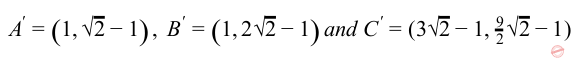BCDEngineering-Mathematics       Co-ordinate-Geometry
Question 14 Explanation: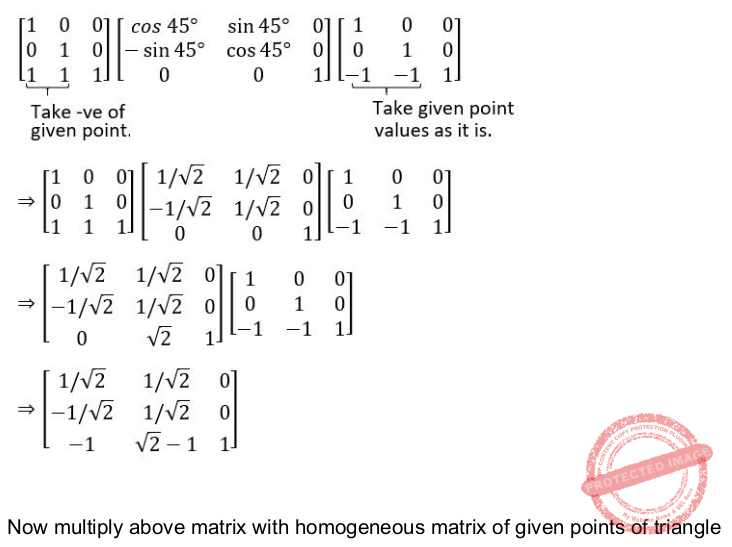Question 15
The process of dividing an analog signal into a string of discrete outputs, each of constant amplitudes, is called:
 A Strobing B Amplification C Conditioning D Quantization
Computer-Networks       Signals
Question 15 Explanation:
In analog to digital signal conversion following steps are performed: 1. Sampling: The analog signal is sampled every Ts seconds, where Ts is the sample interval or period. The result is a sequence of samples that retains the shape of the analog signal.
2. Quantization: The sampled signal is quantized. The result of sampling is a series of pulses with amplitude values between the maximum and minimum amplitudes of the signal. The set of amplitudes can be infinite with non-integral values between the two limits.
So quantization is the process of mapping a large set of input values to a smaller(countable) set.
3. Encoding: After each sample is quantized and the number of bits per sample is decided. The quantized values are encoded as streams of bits.
 Question 16
Which of the following is not a basic primitive of the Graphics Kernel System (GKS)?
 A POLYLINE B POLYDRAW C FILL AREA D POLYMARKER
Computer-Organization       Graphics
Question 16 Explanation:
Graphics Kernel System (GKS): ​ GKS provides a set of drawing features for two-dimensional vector graphics suitable for charting and similar duties. The calls are designed to be portable across different programming languages, graphics devices and hardware, so that applications written to use GKS will be readily portable to many platforms and devices.
The five main primitives in GKs are :
● Polyline: ​ which draws a sequence of connected line segments.
● Fill Area: which displays a specified area.
● Cell array: which displays an image composed of a variety of colours or grey scales.
● Polymarker: which marks a sequence of points with the same symbol.
● Text: which draws a string of characters
 Question 17
Which of the following statement(s) is/are incorrect ?
 A Mapping the coordinates of the points and lines that form the picture into the appropriate coordinates on the device or workstation is known as viewing transformation. B The coordinate system that corresponds to the device or workstation where the image is to be displayed is known as physical device coordinate system C The right-handed cartesian coordinates system in whose coordinates we describe the picture is known as world coordinate system. D Left - handed coordinate system in which the display area of the virtual display device corresponds to the unit (|x|) square whose lower left-hand corner is at the origin of the coordinate system, is known as normalized device coordinate system.
Engineering-Mathematics       Co-ordinate-Geometry
 Question 18A (a)-(i), (b)-(ii), (c)-(iii), (d)-(iv), (e)-(v) B (a)-(ii), (b)-(iii), (c)-(i), (d)-(iv), (e)-(v) C (a)-(iii), (b)-(i), (c)-(ii), (d)-(v), (e)-(iv) D (a)-(iv), (b)-(v), (c)-(i), (d)-(ii), (e)-(iii)
Computer-Graphics       Display-System
Question 18 Explanation:
Flood Gun: An electron gun designed to flood the entire screen with electrons.
Collector: Partly energized by flooding gun stores the charge generated by the writing gun.
Ground: Used to discharge the collector.
 Question 19
Minimal deterministic finite automaton for the language L = {0​ n​ | n ≥ 0, n ≠ 4} will have:
 A 1 final state among 5 states B 4 final states among 5 states C 1 final state among 6 states D 5 final states among 6 states
Theory-of-Computation       Finite-Automata
Question 19 Explanation:
L = { ε, 0 , 0 0, 0 00, 0 0000, 0 00000, . ..}Question 20
The regular expression corresponding to the language L where L = { x ε {0, 1}*|x ends with 1 and does not contain substring 00 } is:
 A (1 + 01) * (10 + 01) B (1 + 01) * 01 C (1 + 01) * (1 + 01) D (10 + 01) * 01
Theory-of-Computation       Regular-Expression
Question 20 Explanation:
L = { 1, 01, 11, 111, 001, ............}
Option(A) is not correct because it can generate “10” which is not ending with “1”.
Option(B): It can’t generate “1” which is a string belongs to given language L. So it is not a correct option.
Option(C) : It is the correct option because it can generate all the strings generated by a given language.
Option(D): It is not the correct answer because it can’t generate strings like 1,11,........
 Question 21A q​ 0​ and q​ 0​ respectively B q​ 0​ and q​ 1​ respectively C q​ 0​ and q​ 2​ respectively D q​ 0​ and q​ 3​ respectively
Theory-of-Computation       Finite-Automata
Question 21 Explanation: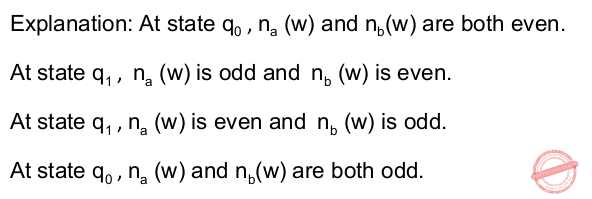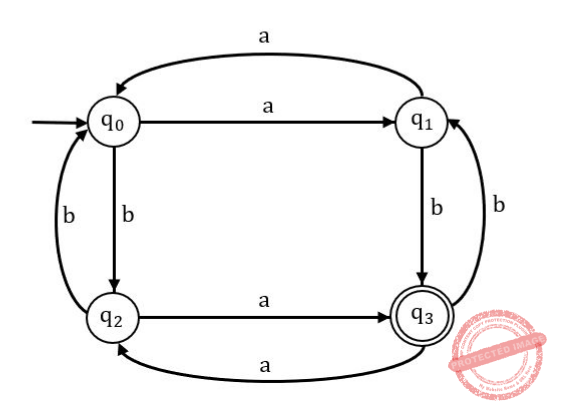Question 22
The clausal form of the disjunctive normal form ¬A ∨ ¬B ∨ ¬C ∨ D is:
 A A ∧ B ∧ C ⇒ D B A ∨ B ∨ C ∨ D ⇒ true C A ∧ B ∧ C ∧ D ⇒ true D A ∧ B ∧ C ∧ D ⇒ false
Engineering-Mathematics       Propositional-Logic
Question 22 Explanation: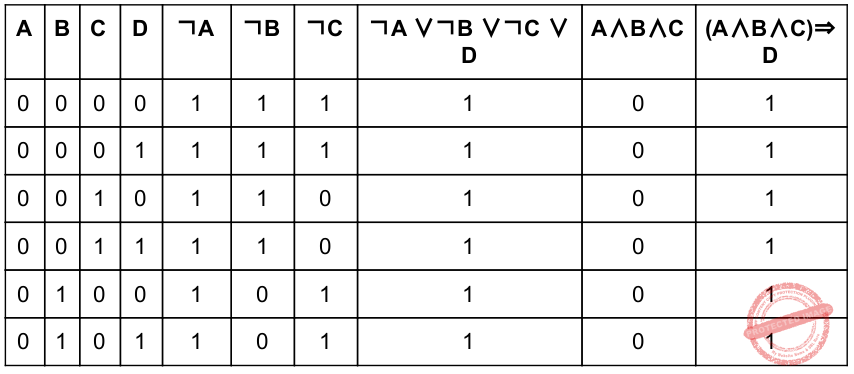Question 23
Which of the following is false for the programming language PROLOG?
 A A PROLOG variable can only be assigned to a value once B PROLOG is a strongly typed language C The scope of a variable in PROLOG is a single clause or rule D The scope of a variable in PROLOG is a single query
Programming       PROLOG
 Question 24
Which one of the following is true?
 A The resolvent of two Horn clauses is not a Horn clause B The resolvent of two Horn clauses is a Horn clause C If we resolve a negated goal G against a fact or rule A to get clause C then C has positive literal or non-null goal D If we resolve a negated goal G against a fact or rule A to get clause C then C has positive literal or null goal
Engineering-Mathematics       Horn-Clause
 Question 25
Which transmission technique guarantees that data packets will be received by the receiver in the same order in which they were sent by the sender?
 A Broadcasting B Unicasting C Packet switching D Circuit switching
Computer-Networks       Transmission-techniques
Question 25 Explanation:
Broadcasting: It is a data transportation method over a network. In this method a source transmit same data to multiple destinations.
Unicasting: It is also a data transportation method over a network. In this method data is transferred from one source to one destination.
Packet Switching: It is a data transmission technique in which data packets make reach the destination using different paths. Hence in this techniques the order of data packets is not guaranteed.
Circuit switching: In this techniques all data packets use the same path to reach the destination. Hence this technique guarantees that data packets will be received by the receiver in the same order in which they were sent by the sender.
 Question 26
Which of the following control fields in the TCP header is used to specify whether the sender has no more data to transmit?
 A FIN B RST C SYN D PSH
Computer-Networks       TCP/IP
Question 26 Explanation:
FIN: FIN flag in the TCP header is used to indicate the connection termination request. It means when a host has no more data to send then it sends the FIN request to its destination to terminate the connection.
RST: RST flag is used to reset the connection.
SYN: SYN flag is used to synchronise/establish a connection.
PSH: PGH flag is used to transmit data immediately without waiting for the buffer to become full.
 Question 27
Which are the two modes of IP security?
 A Transport and certificate B Transport and tunnel C Certificate and tunnel D Pre Shared and transport
Question 27 Explanation:
Transport Mode:​ In this mode security is applied to the transport layer packet and then it is given to IP module to add IPv4 or IPv6 header to this secured packet. It is used to provide security between host to host.
Tunnel Mode:​ In this mode, security is applied to IP layer packet. It is used to provide security between router to router, router to host, gateway to gateway, gateway to host.
 Question 28
A message "COMPUTERNETWORK" encrypted (ignore quotes) using columnar transposition cipher with a key "LAYER". The encrypted message is :
 A CTTOEWMROPNRUEK B MROUEKCTTPNROEW C OEWPNRCTTUEKMRO D UEKPNRMROOEWCTT
Computer-Networks       Network-Security
Question 28 Explanation:Question 29
Suppose a digitized voice channel is made by digitizing 8 kHz bandwidth analog voice signal. It is required to sample the signal at twice the highest frequency (two samples per hertz). What is the bit rate required, if it is assumed that each sample requires 8 bits?
 A 32 kbps B 64 kbps C 128 kbps D 256 kbps
Computer-Networks       Signals
Question 29 Explanation:
t is required to sample the signal at twice the highest frequency (two samples per hertz).
Bit rate = current bandwidth * 2 * sample size.
Bit rate = 8 KHz * 2 * 8 bit.
= 8 K / sec * 2 * 8 bit.
= 128 kb / sec.
= 128 Kbps.
 Question 30
The maximum payload of a TCP segment is:
 A 65,535 B 65,515 C 65,495 D 65,475
Computer-Networks       TCP/IP
Question 30 Explanation:
Total length of the IP header is 16-bits i.e 2​ 16​ = 65,536 bits of data can be sent.65,496 bits means range is 0 - 65,495.
Hence correct answer is option (C).
 Question 31
An all-pairs shortest-paths problem is efficiently solved using:
 A Dijkstra' algorithm B Bellman-Ford algorithm C Kruskal algorithm D Floyd-Warshall algorithm
Algorithms       Dynamic-Programming
Question 31 Explanation:
Dijkstra' algorithm: It ia a greedy algorithm which is used to find the shortest distance between a source to a single destination. It can calculate the shortest path in a graph having no negative weights.
Bellman-Ford algorithm: It is a dynamic programming algorithm used to find the shortest distance between a source to a single destination. It can detect a cycle in a graph having negative weights so in such a graph it do not find the shortest path.
Kruskal algorithm: It is a greedy algorithm which is used to find the minimum spanning tree in a graph.
Floyd-Warshall algorithm: It is a dynamic algorithm which is used to find all-pairs shortest-paths.
 Question 32
The travelling salesman problem can be solved in:
 A Polynomial time using dynamic programming algorithm B Polynomial time using branch-and-bound algorithm C Exponential time using dynamic programming algorithm or branch-and-bound algorithm D Polynomial time using backtracking algorithm
Algorithms       Dynamic-Programming
Question 32 Explanation:
Travelling salesman problem can be solved in Exponential time using dynamic programming algorithm or branch-and-bound algorithm. It’s time complexity is 2​ n​ n​ 2​ .
 Question 33
Which of the following is asymptotically smaller?
 A lg(lg*n) B lg*(lgn) C lg(n!) D lg*(n!)
Algorithms       Asymptotic-Complexity
Question 33 Explanation:
Option-A & B: lg(lg*n) is smaller than lg*(lgn). Because lg*(lg n) = lg*n-1.
Option C and D they are given n!. It means, greater than Option A and B.
 Question 34
Consider a hash table of size m = 100 and the hash function h(k) = floor(m(kA mod 1)) for A = frac{(sqrt5 - 1) 2 = 0.618033 Compute the location to which the key k = 123456 is placed in hash table.
 A 77 B 82 C 88 D 89
Data-Structures       Hashing
Question 34 Explanation:
h(k) = floor(100(123456* 0.618033 mod 1))
= floor(100(0.88204))
= floor(88.204)
= 88
 Question 35
Let f(n) and g(n) be asymptotically nonnegative functions. Which of the following is correct?
 A θ(f(n)*g(n)) = min(f(n), g(n)) B θ(f(n)*g(n)) = max(f(n), g(n)) C θ(f(n)+g(n)) = min(f(n), g(n)) D θ(f(n)+g(n)) = max(f(n), g(n))
Algorithms       Asymptotic-Complexity
Question 35 Explanation: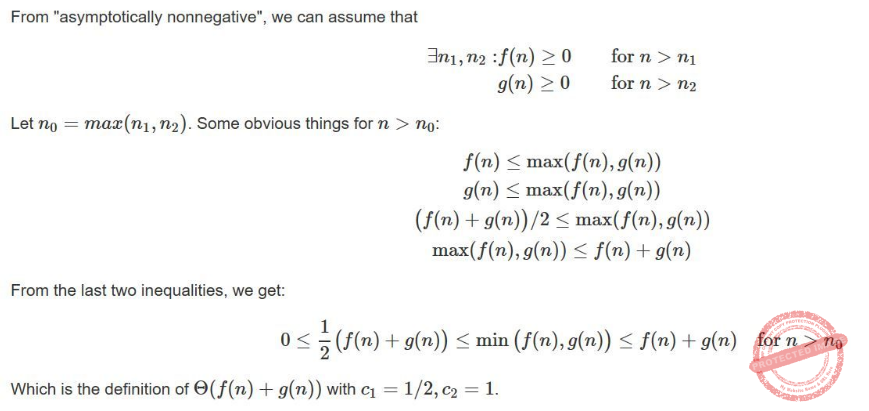Question 36
The number of nodes of height ‘h’ in any n-element heap is __________.
 A h B z​ h C ⌈n/(z​ h​ )⌉ D ⌈n/z​ h+1​ ) E None of the above
Data-Structures       Heap-Tree
Question 36 Explanation:Question 37
In Java, when we implement an interface method, it must be declared as:
 A Private B Protected C Public D Friend
OOPS       JAVA
 Question 38
The Servlet Response interface enables a servlet to formulate a response for a client using the method __________.
 A void log(Exception e, String s) B void destroy( ) C int get ServerPort( ) D void set ContextType(String type)
Computer-Networks       client-server-spplication
 Question 39
Which one of the following is correct?
 A Java applets can not be written in any programming language B An applet is not a small program C An applet can be run on its own D Applets are embedded in another applications
OOPS       JAVA
 Question 40
I​ n XML we can specify the frequency of an element by using the symbols:
 A + * ! B # * ! C +* ? D - * ?
Web-Technologies       XML
 Question 41
In XML, DOCTYPE declaration specifies to include a reference to __________ file.
 A Document type Definition B Document type declaration C Document transfer definition D Document type language
Web-Technologies       XML
 Question 42
Module design is used to maximize cohesion and minimize coupling. Which of the following is the key to implement this rule?
 A Inheritance B Polymorphism C Encapsulation D Abstraction
Software-Engineering       Coupling-and-Cohesion
 Question 43
Verification:
 A refers to the set of activities that ensure that software correctly implements a specific function B gives answer to the question - Are we building the product right ? C requires execution of software D both (A) and (B)
Software-Engineering       Software-configuration-management
Question 43 Explanation:
Verification: It refers to the set of activities that ensure that software correctly implements a specific function.
While coding the programmer verifies that whether the code is working correctly or not.
Verification ensures “Are we building the product right”.
 Question 44
Which design metric is used to measure the compactness of the program in terms of lines of code?
 A Consistency B Conciseness C Efficiency D Accuracy
Software-Engineering       Software-design
Question 44 Explanation:
Consistency: The use of uniform design and documentation techniques throughout the software development project.
Conciseness: used to measure the compactness of the program in terms of lines of code.
Efficiency: It refers to the amount of computing resources and code required by a program to perform its function.
Accuracy: The precision of computations and control.
 Question 45
Requirements prioritisation and negotiation belongs to:
 A Requirements validation B Requirements elicitation C Feasibility study D Requirements reviews
Software-Engineering       Software-requirements
Question 45 Explanation:
Requirement Engineering: ​ The broad spectrum of tasks and techniques that lead to an understanding of requirements is called ​ requirements engineering
Inception: ​ At project inception, you establish a basic understanding of the problem, the people who want a solution, the nature of the solution that is desired, and the effectiveness of preliminary communication and collaboration between the other stakeholders and the software team.
Elicitation : To help overcome problems understanding, volatility, scope, you must approach requirements gathering in an organized manner.
Elaboration ​ This task focuses on developing a refined requirements model that identifies various aspects of software function, behavior, and information..
Negotiation ​ Customers, users, and other stakeholders are asked to rank requirements and then discuss conflicts in priority. Using an iterative approach that prioritizes requirements, assesses their cost and risk, and addresses internal conflicts, requirements are eliminated, combined, and/or modified so that each party achieves some measure of satisfaction.
Specification: A specification can be a written document, a set of graphical models, a formal mathematical model, a collection of usage scenarios, a prototype, or any combination of these.
 Question 46
Adaptive maintenance is a maintenance which __________.
 A correct errors that were not discovered till testing phase B is carried out to port the existing software to a new environment. C improves the system performance D both (2) and (3)
Software-Engineering       Software-configuration-management
Question 46 Explanation:
Correction.: Even with the best quality assurance activities, it is likely that the customer will uncover defects in the software. Corrective maintenance changes the software to correct defects.
Adaptation. Over time, the original environment (e.g., CPU, operating system, business rules, external product characteristics) for which the software was developed is likely to change. Adaptive maintenance results in modifications to the software to accommodate changes to its external environment.
Enhancement/Perfective. As software is used, the customer/user will recognize additional functions that will provide benefit. Perfective maintenance extends the software beyond its original functional requirements.
Prevention. Computer software deteriorates due to change, and because of this, preventive maintenance, often called software reengineering, must be conducted to enable the software to serve the needs of its end users. In essence, preventive maintenance makes changes to computer programs so that they can be more easily corrected, adapted, and enhanced.
 Question 47
A Design concept Refinement is a
 A Top-down approach B Complementary of Abstraction concept C Process of elaboration D All of the above
Software-Engineering       Software-Reliabiliy
Question 47 Explanation:
In the software engineering context, design concept is a top down approach which focuses on four major areas of concern: data, architecture, interfaces, and components.
The ​ data design t ​ ransforms the information domain model created during analysis into the data structures that will be required to implement the software.
The ​ architectural design d ​ efines the relationship between major structural elements of the software.
The ​ interface design d ​ escribes how the software communicates within itself, with systems that interoperate with it, and with humans who use it.
The ​ component-level design t ​ ransforms structural elements of the software architecture into a procedural description of software components.
Hence we can say that design concept is ​ Complementary of Abstraction concept and is a Process of elaboration.
 Question 48
A software design is highly modular if:
 A cohesion is functional and coupling is data type. B cohesion is coincidental and coupling is data type. C cohesion is sequential and coupling is content type. D cohesion is functional and coupling is stamp type.
Software-Engineering       Software-design
Question 48 Explanation:
A good software should have high cohesion and low coupling.
Coupling: It is a measure of the degree of interdependence between modules.Question 49A (a)-(iii), (b)-(i), (c)-(ii), (d)-(iv) B (a)-(iii), (b)-(i), (c)-(iv), (d)-(ii) C (a)-(iv), (b)-(iii), (c)-(ii), (d)-(i) D (a)-(ii), (b)-(iii), (c)-(iv), (d)-(i)
Computer-Organization       Match-the-following
Question 49 Explanation:
Spooling→ Overlapping I/O and computations
Multiprogramming → Allows several jobs in memory to improve CPU utilization
Time Sharing→ Allows many users to share a computer simultaneously by switching processor frequently
Distributed Computing→ Access to shared resources among geographically dispersed computers in a transparent way
 Question 50
Which of the following statements is not true for Multi Level Feedback Queue processor scheduling algorithm?
 A Queues have different priorities B Each queue may have different scheduling algorithm C Processes are permanently assigned to a queue D This algorithm can be configured to match a specific system under design
Operating-Systems       Process-Scheduling
Question 50 Explanation:
MultiLevel Feedback queue:
● There are more than one queues and each queue have assigned a different priority.
● Since there are more than one queue, therefore more than one scheduling algorithm is applied.
● Job starts in the highest priority queue.
● If time slice expires, drop the job by one level and if time slice does not expire push the job up by one level.
● This algorithm can be configured to match a specific system under design
 Question 51
What is the most appropriate function of Memory Management Unit (MMU)?
 A It is an associative memory to store TLB B It is a technique of supporting multiprogramming by creating dynamic partitions C It is a chip to map virtual address to physical address D It is an algorithm to allocate and deallocate main memory to a process
Operating-Systems       Memory-Management
Question 51 Explanation:
Memory Management Unit (MMU): It is a chip to map virtual address to physical address.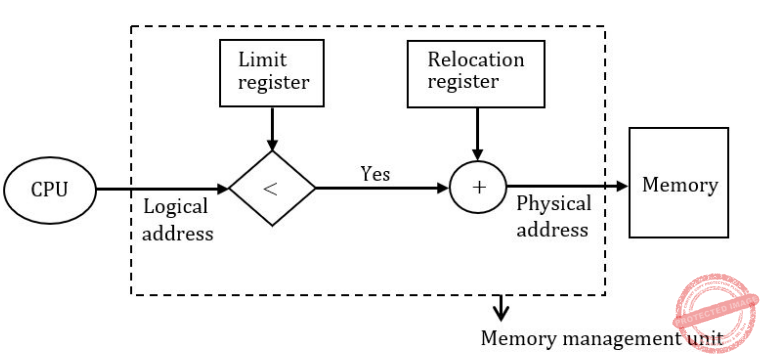Question 52
Dining Philosophers problem is a:
 A Producer - consumer problem B Classical IPC problem C Starvation problem D Synchronization primitive
Operating-Systems       Process-Synchronization
Question 52 Explanation:
Dining Philosophers problem is a Classical IPC(inter process communication) problem.
 Question 53
In __________ allocation method for disk block allocation in a file system, insertion and deletion of blocks in a file is easy.
 A Index B Linked C Contiguous D Bitmap
Operating-Systems       File system
Question 53 Explanation:
Linked allocation is easy to implement in comparison to other allocation methods. In linked allocation we have to change pointers only and because of this disk block allocation in a file system, insertion and deletion of blocks in a file is easy.
 Question 54
A unix file may be of the type:
 A Regular file B Directory file C Device file D Any one of the above
Operating-Systems       UNIX-Operating-System
Question 54 Explanation:
Regular(Ordinary) file: ​ Ordinary files contain ASCII (human-readable) text, executable program binaries, program data, and more.
Directory File: A directory file contains an entry for all files or subdirectories that it contains.
Special(device) file: It contains information about all the devices connected to the system.
 Question 55A (a)-(iii), (b)-(iv), (c)-(i), (d)-(ii) B (a)-(iii), (b)-(iv), (c)-(ii), (d)-(i) C (a)-(iv), (b)-(iii), (c)-(i), (d)-(ii) D (a)-(iv), (b)-(iii), (c)-(ii), (d)-(i)
Artificial-intelligence       Knowledge-representation
Question 55 Explanation:
Intelligence → Judgemental
Knowledge → Codifiable, endorsed with relevance and purpose
Information → Scattered facts, easily transferable
Data → Contextual, tacit, transfer needs learning
 Question 56A (a)-(iii), (b)-(iv), (c)-(i), (d)-(ii) B (a)-(iii), (b)-(iv), (c)-(ii), (d)-(i) C (a)-(iv), (b)-(iii), (c)-(i), (d)-(ii) D (a)-(iv), (b)-(iii), (c)-(ii), (d)-(i)
Computer-Science-General-Aptitude       Computer-Science-Basics
Question 56 Explanation:
Frames → Record like structures for grouping closely related knowledge
Conceptual → Structures and primitives to represent sentences
Associative networks → Pictorial representation of objects, their attributes and relationships
Scripts → To describe real world stereotype dependencies events
 Question 57
In propositional logic P ↔ Q is equivalent to (Where ~ denotes NOT):
 A ~( P ∨ Q ) ∧ ~ ( Q ∨ P ) B ( ~P ∨ Q ) ∧ (~ Q ∨ P ) C ( P ∨ Q ) ∧ ( Q ∨ P ) D ~( P ∨ Q ) → ~ ( Q ∨ P )
Engineering-Mathematics       Propositional-Logic
Question 57 Explanation: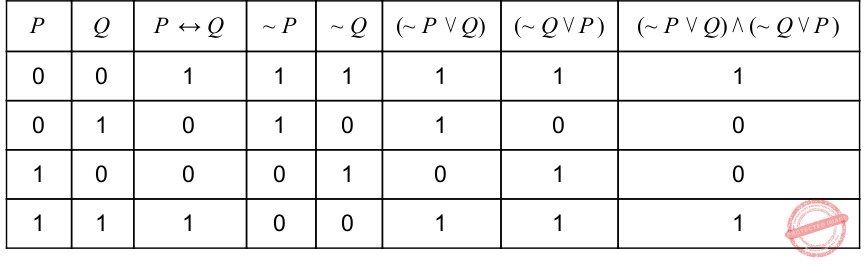Question 58
Which of the following statements is true for Branch - and - Bound search?
 A Underestimates of remaining distance may cause deviation from optimal path. B Overestimates can't cause right path to be overlooked. C Dynamic programming principle can be used to discard redundant partial paths. D All of the above E None of these
Algorithms       Branch-and-Bound
 Question 59A (a)-(i), (b)-(iv), (c)-(iii), (d)-(ii) B (a)-(iv), (b)-(i), (c)-(ii), (d)-(iii) C (a)-(i), (b)-(iv), (c)-(ii), (d)-(iii) D (a)-(iv), (b)-(ii), (c)-(i), (d)-(iii)
Artificial-intelligence       Hill-Climbing-Algorithm
Question 59 Explanation:
Steepest – accent Hill Climbing→ Considers all moves from current state and selects best move.
Branch – and – bound → Keeps track of all partial paths which can be a candidate for further exploration
Constraint satisfaction → Discover problem state(s) that satisfy a set of constraints
Means – end – analysis → Detects difference between current state and goal state
 Question 60A (a)-(i), (b)-(iii), (c)-(ii), (d)-(iv) B (a)-(iv), (b)-(i), (c)-(ii), (d)-(iii) C (a)-(i), (b)-(v), (c)-(ii), (d)-(iii) D (a)-(iv), (b)-(ii), (c)-(i), (d)-(iii)
Software-Engineering       Software-design
Question 60 Explanation:
Joint Application Design → A group based tool for collecting user requirements and creating system design. Mostly used in analysis and design stages of SDLC.
Computer Aided Software Engg → Delivers functionality in rapid iterations measured in weeks and needs frequent communication, development, testing and delivery.
Agile → Reusable applications generally with one specific function. It is closely linked with the idea of web services and service oriented architecture.
Component based technology → Tools to automate many tasks of development SDLC.
 Question 61
A context free grammar for L = { w | n​ 0​ ( w ) > n​ 1​ ( w ) } is given by:
 A S → 0 | 0 S | 1 S S B S → 0 S | 1 S | 0 S S | 1 S S | 0 | 1 C S → 0 | 0 S | 1 S S | S 1 S | S S 1 D S → 0 S |1 S | 0 | 1
Theory-of-Computation       Context-Free-Grammar
Question 61 Explanation:
In given language L the number of 0’s should be greater than the number of 1’s.
L= { 0, 00, 000, 100, 001, 010,...........}
Option(A) is incorrect because can’t generate string “001”
Option(B) is incorrect because it is generating string”1” in which number of 0’s is less than number of 1’s.
Option(C) is correct because it can generate all the strings generated by the given language L.
Option(D) is incorrect because it is generating string”1” in which number of 0’s is less than number of 1’s.
 Question 62
Given the following two statements:
S​ 1​ :If L​ 1​ and L​ 2​ are recursively enumerable languages over ∑, then L​ 1​ ∪ L​ 2​ and L​ 2​ ∩ L​ 2​ are also recursively enumerable.
S​ 2​ : The set of recursively enumerable languages is countable. Which of the following is correct ?
 A S​ 1​ is correct and S​ 2​ is not correct B S​ 1​ is not correct and S​ 2​ is correct C Both S​ 1​ and S​ 2​ are not correct. D Both S​ 1​ and S​ 2​ are not correct.
Theory-of-Computation       Recursive-and-Recursively-Enumerable-Language
Question 62 Explanation:
Statement S​ 1​ ​ is true because recursively enumerable language are closed under union and intersection.
Statement S​ 2​ is true because recursively enumerable languages are those languages for which we can have a Turing machine but we can’t say whether the Turing machine will halt on some input or not. Since the set of all Turing machines is countable, hence set of all recursively enumerable languages is also countable.
 Question 63
Given the following grammars:
G​ 1​ : ​ S → AB|aaB
A → aA | ∈
B → bB | ∈
G2: S → A|B
A → aAb | ab
B → abB | ∈
Which of the following is correct?
 A G ​ 1​ is ambiguous and G ​ 2​ is unambiguous grammars B G ​ 1​ is unambiguous and G ​ 2​ is ambiguous grammars C both G ​ 1​ and G ​ 2​ are ambiguous grammars D both G ​ 1​ and G ​ 2​ are unambiguous grammars
Theory-of-Computation       Context-Free-Grammar
Question 63 Explanation:
G1 is ambiguous because for generating string string “aa” we are having two different leftmost trees.G2 is ambiguous because for generating string ab we are having two different leftmost trees.NOTE: A grammar is ambiguous only if it can generate two different trees(either leftmost derivation tree or rightmost derivation tree) for a same string.
 Question 64
Given the symbols A, B, C, D, E, F, G and H with the probabilities 1/30, 1/30, 1/30, 2/30, 3/30, 5/30, 5/30, and 12/30 respectively. The average Huffman code size in bits per symbol is:
 A 67/30 B 70/30 C 76/30 D 78/30 E None of the above
Algorithms       Greedy-approach
Question 64 Explanation: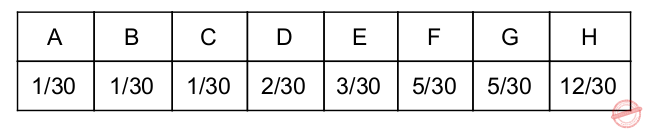Question 65
The redundancy in images stems from:
 A pixel decorrelation B pixel correlation C pixel quantization D image size
Computer-Organization       Graphics
Question 65 Explanation:
The redundancy in images stems from pixel correlation
 Question 66
In a binary Hamming Code the number of check digits is r then number of message digits is equal to:
 A 2​ r​ - 1 B 2​ r​ - r - 1 C 2​ r​ - r + 1 D 2​ r​ + r - 1
Digital-Logic-Design       Number-Systems
Question 66 Explanation:
2 p = m + p + 1 ---(1)
where p is the no. of parity bits.
m = no. of msg. digits
No. of check bits in a msg = p
In question no. of check-bits are given as ‘r’
So r = p
Put it in equation (1)
2 r = m + r + 1
m = 2 r − r − 1
 Question 67
In the Hungarian method for solving assignment problem, an optimal assignment requires that the maximum number of lines that can be drawn through squares with zero opportunity cost is equal to the number of:
 A rows or columns B rows + columns C rows + columns - 1 D rows + columns + 1
LPP       Assignment-Problem
Question 67 Explanation:
In the Hungarian method for solving assignment problem, an optimal assignment requires that the maximum number of lines that can be drawn through squares with zero opportunity cost is equal to the number of rows or columns.
 Question 68
Consider the following transportation problem: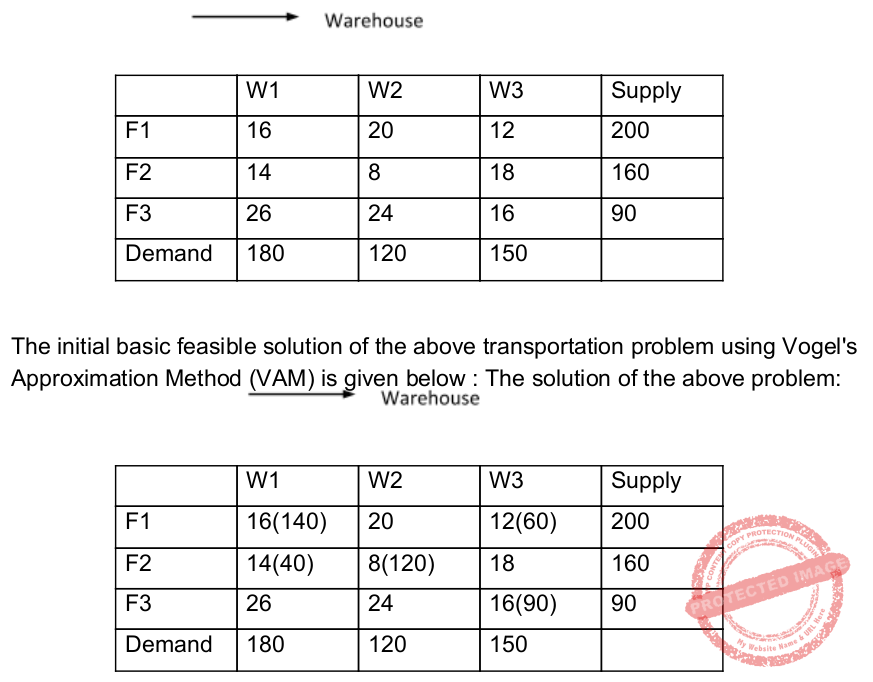A is degenerate solution B is optimum solution C needs to improve D is infeasible solution
LPP       Transportation-Problem
Question 68 Explanation:
Step1: In vogel’s approximation method first find out the row difference(by calculating the difference between the two smallest values of that row) and column difference(by calculating the difference between the two smallest values of that row)
Then select the row having highest row difference and after selecting row choose the column/cell of that row having minimum value.
After that fulfill the demand by using supply from the selected row and column.
After that if demand becomes zero then delete that column and if the row become zero then delete that row and then repeat step 1.Question 69
Given the following statements with respect to linear programming problem:
S​ 1​ : The dual of the dual linear programming problem is again the primal problem
S​ 2​ : If either the primal or the dual problem has an unbounded objective function value, the other problem has no feasible solution.
S​ 3​ : If either the primal or dual problem has a finite optimal solution, the other one also possesses the same, and the optimal value of the objective functions of the two problems are equal.
Which of the following is true?
 A S​ 1​ and S​ 2 B S​ 1​ and S​ 3 C S​ 2​ and S​ 3 D S​ 1​ , S​ 2​ and S​ 3
LPP       Duality
Question 69 Explanation:
S​ 1​ : TRUE: The dual of the dual linear programming problem is again the primal problem
S​ 2​ : TRUE: If either the primal or the dual problem has an unbounded objective function value, the other problem has no feasible solution.
S​ 3​ : TRUE: If either the primal or dual problem has a finite optimal solution, the other one also possesses the same, and the optimal value of the objective functions of the two problems are equal.
 Question 70
Let A and B be two fuzzy integers defined as :
A = {(1, 0.3), (2, 0.6), (3, 1), (4, 0.7), (5, 0.2)}
B = {(10, 0.5), (11, 1), (12, 0.5)}
Using fuzzy arithmetic operation given byA {(11, 0.8), ,(13, 1), (15, 1)} B {(11, 0.3), ,(12, 0.5), (13, 1), (14, 1), (15, 1), (16, 0.5), (17, 0.2)} C {(11, 0.3), ,(12, 0.5), (13, 0.6), (14, 1), (15, 1), (16, 0.5), (17, 0.2)} D {(11, 0.3), ,(12, 0.5), (13, 0.6), (14, 1), (15, 0.7), (16, 0.5), (17, 0.2)}
Engineering-Mathematics       Fuzzy-logic
Question 70 Explanation:Step 2: Now from Step 1 select distinct elements and if two elements are same then select the one having maximum membership.
μ A+B (2) = { (11, 0 .3) (12, 0 .5) (13, 0 .6) (14, 1 ) (15, 0 .7) (16, 0 .5) (17, 0 .2)}
⟶ Hence option (D) is the correct answer.
 Question 71
Suppose the function y and a fuzzy integer number around - 4 for x are given as y = (x - 3)​ 2​ + 2.
Around - 4 = {(2, 0.3), (3, 0.6), (4, 1), (5, 0.6), (6, 0.3)} respectively. Then f (Around - 4) is given by:
 A {(2, 0.6), (3, 0.3), (6, 1), (11, 0.3)} B {(2, 0.6), (3, 1), (6, 1), (11, 0.3)} C {(2, 0.6), (3, 1), (6, 0.6), (11, 0.3)} D {(2, 0.6), (3, 0.3), (6, 0.6), (11, 0.3)}
Engineering-Mathematics       Fuzzy-logic
Question 71 Explanation:⟶ Take highest membership i.e., ‘1’.
So Around -4 for y is
{(3, 1 ) (2, 0 .6) (6, 0 .6) (11, 0 .3)}
⟶ Hence option (C) is the correct answer.
 Question 72A (a)-(ii), (b)-(iii), (c)-(iv), (d)-(i) B (a)-(iii), (b)-(ii),(c)-(iv), (d)-(i) C (a)-(iv), (b)-(iii), (c)-(ii), (d)-(i) D (a)-(iv), (b)-(iii), (c)-(i), (d)-(ii)
Operating-Systems       System-Calls
Question 72 Explanation:
exec → Invokes another program overlaying memory space with a copy of the executable file
brk → To increase or decrease the size of data region
wait → A process synchronizes with termination of child process
fork → Creates a new process
 Question 73
WOW32 is a:
 A Win 32 API library for creating processes and threads. B Special kind of file system to the NT namespace. C Kernel - mode objects accessible through Win 32 API D Special execution environment used to run 16 bit Windows applications on 32 - bit machines.
Operating-Systems       win32
Question 73 Explanation:
WOW32 is a Special execution environment used to run 16 bit Windows applications on 32 - bit machines.
 Question 74
The unix command: \$vi file1 file2
 A Edits file1 and stores the contents of file1 in file2 B Both files i.e. file1 and file2 can be edited using 'ex' command to travel between the files C Both files can be edited using 'mv' command to move between the files D Edits file1 first, saves it and then edits file2
Operating-Systems       UNIX-Operating-System
Question 74 Explanation:
\$vi file1 file2 → Both files i.e. file1 and file2 can be edited using 'ex' command to travel between the files.
There are 74 questions to complete.